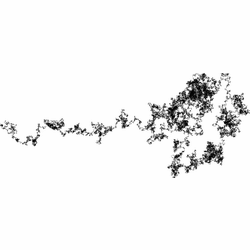# Zoom on a Random Walk

After reading Darren's post about the Mandelbrot set I was browsing through the Wikipedia entry on the Mandelbrot set which has a few amazing animations of really deep zooms on the fractal bounded by the set.

The good/bad thing about fractals is that as you magnify them, the pattern and resulting image that you see is in some way similar to patterns you saw at lower magnifications. So the zooms are in some sense self-similar.

It occurred to me that viewing random walks at different magnifications should have a similar property. You should not be able to tell what magnification you are looking at, since the average trajectory should be random, regardless of the scale of the trajectory. As long as you are away from the scale at which you can observe any discrete steps making up the walk, that is.

So, we should be able to make semi-infinite zooms of random walks, in which all magnifications look believably random and as if they were generated from the same process but without any repeating or recurring patterns. I think the random walks are quite pleasing to look at. It is a bit tricky to create this zoom though, since I can't think of any cunning way to step from one magnification to another by "filling in" the gaps in the walk that have just come into view. So I have to create a massive random walk image, and progressively resize and crop it to achieve the zoom. Also, the PyGame code I posted a while ago is too slow for generating a massive random walk. However, there's no need to see the walk being generated in this case, so we can just colour pixels black and anti-alias during image resize (using the Python Imaging Library) instead of doing it in real-time.

Deep zooming is cool anyway as you can see in the amazing Powers of Ten video by Charles and Ray Eames.

So, here's a zoom on a random walk that I made (approximately 100 times zoom, made on a 32-bit machine with 3.5Gb RAM):The frames were generated with the Python code below. If you have a more powerful computer than me to hand, you can generate a deeper zoom! Just increase the width and height parameters until you run out of memory or patience. I patched the output images together and created a .gif animation using the amazing VirtualDub.

```import PIL,random,math,os
from PIL import Image,ImageDraw

# Define screen size and initialize
size=width,height=3500,3500
plot=Image.new("L",(width,height),'white')

# Output Frame size
wf,hf=250,250
# Zoom parameters
zmax,zno=1.0,700

# Start at centre of image
x,y=width/2,height/2
pix[x,y]=0

# Prepare to find the bounding box for the walk
xmin,ymin,xmax,ymax=x,y,x,y
pcount=0

# Start walking until we hit the edge
while 1<=x<width-1 and 1<=y<height-1:
# Generate new steps
xnew=x+random.randint(-1,1)
ynew=y+random.randint(-1,1)
x,y=xnew,ynew
pix[x,y]=0
pcount+=1
if x<xmin:xmin=x
if x>xmax:xmax=x
if y<ymin:ymin=y
if y>ymax:ymax=y

# Crop to the walk
plot=plot.crop((xmin,ymin,xmax,ymax))

# Rotate if necessary to make image wider than tall
if xmax-xmin<ymax-ymin:
plot=plot.rotate(90)
(w,h)=plot.size

# Set minium zoom to just capture entire walk
zmin=float(wf)/float(w)

# For geometric zooming:
# zoom(z) = a*pow(phi,b*z)
# insist that zoom(0)=zmin, zoom(zno)=zmax
phi=10.0
a=zmin
b=math.log(zmax/zmin,phi)/zno

for z in xrange(0,zno+1):
zoom=a*pow(phi,b*z)
print "FRAME: ",z, zoom
blank=Image.new("L",(wf,hf),'white')
if z>0 and z%50==0:
# Throw away pixels to achieve deeper zoom with set memory
cz=float(hf)/float(hnew)
xa=max(0,int(round(float(w)/2.0-cz*float(w)/2.0)))
ya=max(0,int(round(float(h)/2.0-cz*float(h)/2.0)))
xb=min(w,int(round(float(w)/2.0+cz*float(w)/2.0)))
yb=min(h,int(round(float(h)/2.0+cz*float(h)/2.0)))
plot=plot.crop((xa,ya,xb,yb))
(w,h)=plot.size
wnew=int(round(zoom*float(w)))
hnew=int(round(zoom*float(h)))
# Resize the frame
small=plot.resize((wnew+1,hnew+1),Image.ANTIALIAS)
# This crop is to trim grey lines which appear
small=small.crop((1,1,wnew+1,hnew+1))
xmin=int(round(float(wnew)/2.0-float(wf)/2.0))
ymin=int(round(float(hnew)/2.0-float(hf)/2.0))
xmax=int(round(float(wnew)/2.0+float(wf)/2.0))
ymax=int(round(float(hnew)/2.0+float(hf)/2.0))
if wnew<wf or hnew<hf:
frame=Image.new("L",(wf,hf),'white')
px=int(round(float(wf-wnew)/2.0))
py=int(round(float(hf-hnew)/2.0))
frame.paste(small,(px,py))
else:
frame=small.crop((xmin,ymin,xmax,ymax))
fname="FrameL%05d.png"%z
frame.save(fname)

print "Zoom: ",1.0/zmin
print "Steps: ",pcount```